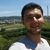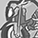Announcements
You can succeed best and quickest by helping others to succeed. Join the conversation.
cancel
Showing results for
Did you mean:Creator II

## Filter Single Value to display

Within my Qlik sheet/Table object

I need to show a single refund value based on filters.

Example: =If(Bank_At_Flag = 'RFND', if(Paid_Amt <= 10.00, SUM(Paid_Amt)))

All I get in return is > - <.

Using Qlik Sense browser based tool.

Thanks for the help!

B

2 Solutions

Accepted SolutionsSpecialist III

SUM({<Bank_At_Flag = {'RFND'}, Paid_Amt  = {'<=10.00'}>}Paid_Amt)

This expression sums up all the paid amount where  Bank_At_Flag = 'RFND' with 'Paid_Amt<=10.00'

Hope that helps.

KCreator II

Hi,

Try:

``SUM({<Bank_At_Flag = {'RFND'}, Paid_Amt  = {'<=10.00'}>} X_PAID_AMT)``

Regards

7 RepliesCreator II

Try this

=if(Paid_Amt <= 10.00, SUM({<Bank_At_Flag = {'RFND'}>}Paid_Amt))Specialist III

SUM({<Bank_At_Flag = {'RFND'}, Paid_Amt  = {'<=10.00'}>}Paid_Amt)

This expression sums up all the paid amount where  Bank_At_Flag = 'RFND' with 'Paid_Amt<=10.00'

Hope that helps.

KCreator II
Author
=SUM({}X_PAID_AMT)

I always get 0.

FYI: Validated with SQL my total should be (\$1,258)Creator II
Author
=if(X_PAID_AMT <= 10.00, Sum({}X_PAID_AMT))

I always get -.

FYI: Validated with SQL my total should be (\$1,258)Creator II

Hi,

Try:

``SUM({<Bank_At_Flag = {'RFND'}, Paid_Amt  = {'<=10.00'}>} X_PAID_AMT)``

RegardsCreator II
Author

That is it!! 🙂   Thank You!Creator II
Author